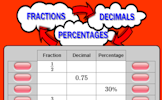# Using percentages

### Term 2 starting in week 3 :: Estimated time: 2 weeks

• Use the equivalence of fractions, decimals and percentages (review)
• Calculate percentage increase and decrease (review)
• Express a change as a percentage (review)
• Solve 'reverse' percentage problems
• Recognise and solve percentage problems (non-calculator)
• Recognise and solve percentage problems (calculator) (review)

For higher-attaining pupils:

• Solve problems with repeated percentage change

This page should remember your ticks from one visit to the next for a period of time. It does this by using Local Storage so the information is saved only on the computer you are working on right now.

## Lesson Starters

Here are some suggestions for whole-class, projectable resources which can be used at the beginnings of each lesson in this block.

### 1st Lesson#### PercenTable

Complete the table by calculating common percentages without using a calculator.

### 2nd Lesson#### Quick Percentages

Simple percentage questions appear on screen then fade every 8 seconds. This Starter is customisable.

### 3rd Lesson#### Fractions Decimals Percentages

Convert fractions to decimals, decimals to percentages and percentages to fractions.

### 4th Lesson#### Noughts and Crosses

In how many different ways can the first X and O by placed on the grid?

### 5th Lesson#### Student Loans

Six students borrow £5000 to help pay for their university course. Calculate how much each student will have to pay back to the lender.

### 6th Lesson#### Render Digitful

Find a calculation for the current year which uses all of the digits 1 to 9.

Some of the Starters above are to reinforce concepts learnt, others are to introduce new ideas while others are on unrelated topics designed for retrieval practice or and opportunity to develop problem-solving skills.

White Rose ResourcesEnd of block assessments provide a quick progress check at the end of each block of learning to make sure students have understood the content covered. This Scheme of Learning was produced by White Rose Maths and is used here with permission granted on 30th June 2021.For All: